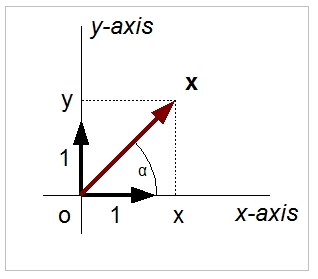# Vector Quantities

A vector is defined as:

x = (x, y, z)

where:
x = position on the x axis
y = position on the y axis
z = position on the z axis

Note that the scalar values x, y, z refer to the unit vectors (with a length of 1) along the x, y and z axes in Cartesian space. On this website, vectors are denoted by bold typeface.

The magnitude (or length) of the vector (x, y, z) is: sqrt(x2 + y2 + z2)

The direction can be expressed by the angles α and β with:

α = arctan (y / x)
β = arctan (z / x)

The vector cross product is defined as:

a x b = (a2b3 - a3b2, a3b1 - a1b3, a1b2 - a2b1)

where:
a = (a1, a2, a3
b = (b1, b2, b3

## Explanation

Many quantities have both a magnitude and a direction. The magnitude and direction can be expressed by a vector. These vectors are defined by their components along an orthogonal axis system (Cartesian space). Each axis represents a dimension. For example, when we look at the speed and direction of an object, we can denote this by specifying the speed's horizontal component (one leg of the axis system) and its vertical component (the second axis). This is an example of a two dimensional vector quantity. We can also define a three dimensional vector in a similar way.The cross product between two vectors, a x b, has vector c as a result. This vector is orthogonal to a and b, and has a magnitude that is equal to the area of the parallelogram spawned by them. The sign of c is denoted by the right hand rule. Note that when a and b are orthogonal to each other (parallelogram is, then, a square), the magnitude of  c equals the product of the magnitudes of a and b.

## Example 1

The speed of a helicopter is angled at 45 degrees from the horizontal axis in the x-y plane. The speed is 5 m/s along the horizontal axis. How great is the vertical speed, and what is its absolute value (that is, what is the magnitude of the speed)?
Answer: The vertical speed must be equal to its horizontal speed, as the angle in the x-y plane is 45 degrees. The value of the speed is: sqrt(x2 + y2) = sqrt (25 + 25) = sqrt(50) = 7.07 m/s

## Example 2

The speed of a helicopter is 20 m/s and makes an angle of 15 degrees with the x axis and is flying in the x-y plane. What is the vertical component of the speed?
tan(α) = y / x
tan(15) = y/20
y = 20 tan(15) = 5.36

So, the vertical speed component is 5.36 m/s.

## Example 3

The speed of a helicopter is defined by the vector (10, 20, 0). What is the angle with the x-axis?
Answer: angle = arctan (20/10) = arctan (2) = 1.10714872 (in radials)->  In degrees: (1.10714872 / 2pi) * 360 = 63.4 degrees.

# Do you want to comment this topic?

1: (Book) Cyclic and Collective2: (Book) Principles of Helicopter Flight3: Microsoft FSX Steam Edition4: Logitech Extreme 3D Pro Joystick5: Saitek Pro Flight Rudder Pedals